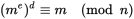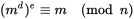# [Solved] What is modulus?

There was a section in ovpn file called “modulus”. What is it in general? I’ve seen this word already when I configured SSL for e-mail server.

In computing, the modulo operation finds the remainder after division of one number by another (sometimes called modulus). Given two positive numbers, a (the dividend) and n (the divisor), a modulo n (abbreviated as a mod n) is the remainder of the Euclidean division of a by n.

This is used in for example RSA.

The basic principle behind RSA is the observation that it is practical to find three very large positive integers e, d and n such that with modular exponentiation for all m:and that even knowing e and n or even m it can be extremely difficult to find d.

Additionally, for some operations it is convenient that the order of the two exponentiations can be changed and that this relation also implies:Thank you for the explanation. It is a bit more clear to me now. I need to get into this math from wiki to understand better. So basically modulus impacts on encryption “difficulty”. Is that correct? Sorry for probably not very smart question. Trying to set it up in my head yet.

Not quite. It simply finds the remainder after the division of one number by another. The remainder is a variable required for the RSA algorithm to work.